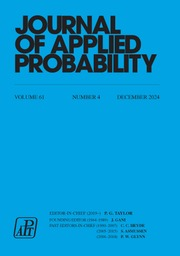Home
Hostname: page-component-79b67bcb76-vkbph Total loading time: 0.229 Render date: 2021-05-12T23:32:36.288Z Has data issue: true Feature Flags: { "shouldUseShareProductTool": true, "shouldUseHypothesis": true, "isUnsiloEnabled": true, "metricsAbstractViews": false, "figures": false, "newCiteModal": false, "newCitedByModal": true, "newEcommerce": true }Journal of Applied Probability

# Stochastic LU factorizations, Darboux transformations and urn models

Published online by Cambridge University Press:  16 November 2018

Corresponding

## Abstract

We consider upper‒lower (UL) (and lower‒upper (LU)) factorizations of the one-step transition probability matrix of a random walk with the state space of nonnegative integers, with the condition that both upper and lower triangular matrices in the factorization are also stochastic matrices. We provide conditions on the free parameter of the UL factorization in terms of certain continued fractions such that this stochastic factorization is possible. By inverting the order of the factors (also known as a Darboux transformation) we obtain a new family of random walks where it is possible to state the spectral measures in terms of a Geronimus transformation. We repeat this for the LU factorization but without a free parameter. Finally, we apply our results in two examples; the random walk with constant transition probabilities, and the random walk generated by the Jacobi orthogonal polynomials. In both situations we obtain urn models associated with all the random walks in question.

## MSC classification

Type
Research Papers
Information
Journal of Applied Probability , September 2018 , pp. 862 - 886

## Access options

Get access to the full version of this content by using one of the access options below.

## References

Castro, M. M. and Grünbaum, F. A. (2013). On a seminal paper by Karlin and McGregor. SIGMA 9, 020.Google Scholar
Chihara, T. S. (1978). An Introduction to Orthogonal Polynomials. Gordon and Breach, New York.Google Scholar
Dette, H., Reuther, B., Studden, W. J. and Zygmunt, M. (2006). Matrix measures and random walks with a block tridiagonal transition matrix. SIAM J. Matrix Anal. Appl. 29, 117142.CrossRefGoogle Scholar
Feller, W. (1968). An Introduction to Probability Theory and Its Applications, Vol. 1, 3rd edn. John Wiley, New York.Google Scholar
Grassmann, W. K. (1987). Means and variances of time averages in Markovian environments. Europ. J. Operat. Res. 31, 132139.CrossRefGoogle Scholar
Grassmann, W. K. (1993). Means and variances in Markov reward systems. In Linear Algebra, Markov Chains and Queueing Models, Springer, New York, pp. 193204.CrossRefGoogle Scholar
Grünbaum, F. A. (2008). Random walks and orthogonal polynomials: some challenges. In Probability, Geometry and Integrable Systems (Math. Sci. Res. Inst. Publ. 55), Cambridge University Press, pp. 241260.Google Scholar
Grünbaum, F. A. (2010). An urn model associated with Jacobi polynomials. Comm. App. Math. Comput. Sci. 5, 5563.CrossRefGoogle Scholar
Grünbaum, F. A. (2011). The Darboux process and a noncommutative bispectral problem: some explorations and challenges. In Geometric Aspects of Analysis and Mechanics (Progr. Math. 292), Birkhäuser, New York, pp. 161177.CrossRefGoogle Scholar
Grünbaum, F. A. and Haine, L. (1996). Orthogonal polynomials satisfying differential equations: the role of the Darboux transformation. In Symmetries and Integrability of Difference Equations, American Mathematical Society, Providence, RI, pp. 143154.CrossRefGoogle Scholar
Grünbaum, F. A., Haine, L. and Horozov, E. (1999). Some functions that generalize the Krall-Laguerre polynomials. J. Comput. Appl. Math. 106, 271297.CrossRefGoogle Scholar
Grünbaum, F. A., Pacharoni, I. and Tirao, J. (2013). Two stochastic models of a random walk in the U(n)-spherical duals of U(n+1). Ann. Mat. Pura Appl. (4) 192, 447473.CrossRefGoogle Scholar
Heyman, D. P. (1995). A decomposition theorem for infinite stochastic matrices. J. Appl. Prob. 32, 893901.CrossRefGoogle Scholar
Karlin, S. and McGregor, J. L. (1957). The differential equations of birth-and-death processes, and the Stieltjes moment problem. Trans. Amer. Math. Soc. 85, 489546.CrossRefGoogle Scholar
Karlin, S. and McGregor, J. (1957). The classification of birth and death processes. Trans. Amer. Math. Soc. 86, 366400.CrossRefGoogle Scholar
Karlin, S. and McGregor, J. (1959). Random walks. IIlinois J. Math. 3, 6681.Google Scholar
Matveev, V. B. and Salle, M. A. (1979). Differential-difference evolution equations II. Darboux transformation for the Toda lattice. Lett. Math. Phys. 3, 425429.CrossRefGoogle Scholar
Spiridonov, V. and Zhedanov, A. (1999). Self-similarity, spectral transformations and orthogonal and biorthogonal polynomials. In Self-Similar Systems, Joint Institute of Nuclear Research, Dubna, pp. 349361.Google Scholar
Vigon, V. (2013). LU-factorization versus Wiener-Hopf factorization for Markov chains. Acta Appl. Math. 128, 137.CrossRefGoogle Scholar
Wall, H. S. (1948). Analytic Theory of Continued Fractions. Van Nostrand, New York.Google Scholar
Yoon, G. J. (2002). Darboux transforms and orthogonal polynomials. Bull. Korean Math. Soc. 39, 359376.CrossRefGoogle Scholar
Zhedanov, A. (1997). Rational spectral transformations and orthogonal polynomials. J. Comput. Appl. Math. 85, 6786.CrossRefGoogle Scholar

# Send article to Kindle

Note you can select to send to either the @free.kindle.com or @kindle.com variations. ‘@free.kindle.com’ emails are free but can only be sent to your device when it is connected to wi-fi. ‘@kindle.com’ emails can be delivered even when you are not connected to wi-fi, but note that service fees apply.

Find out more about the Kindle Personal Document Service.

Stochastic LU factorizations, Darboux transformations and urn models
Available formats
×

# Send article to Dropbox

To send this article to your Dropbox account, please select one or more formats and confirm that you agree to abide by our usage policies. If this is the first time you use this feature, you will be asked to authorise Cambridge Core to connect with your <service> account. Find out more about sending content to Dropbox.

Stochastic LU factorizations, Darboux transformations and urn models
Available formats
×

# Send article to Google Drive

To send this article to your Google Drive account, please select one or more formats and confirm that you agree to abide by our usage policies. If this is the first time you use this feature, you will be asked to authorise Cambridge Core to connect with your <service> account. Find out more about sending content to Google Drive.

Stochastic LU factorizations, Darboux transformations and urn models
Available formats
×
×